HLIBpro  2.3.1
Class Hierarchy
This inheritance list is sorted roughly, but not completely, alphabetically:
[detail level 12345]
 Ccomplex Class for a complex numerical type CTGeomCTBuilder::data_t Datatype for internal argument transfer Cfac_options_t Options for matrix factorisations Chlib_acc_blocked_t Chlib_acc_fixed_eps_t Chlib_acc_fixed_rank_t Chlib_acc_u Cinv_options_t Options for matrix inversion Cis_complex_type< T > Cis_float< T > Cis_integer< T > CTNodeSet::iterator STL iterator for TNodeSet ►CMatrixBase< T_derived > Defines basic interface for matrices ►CMatrixBase< AdjoinView< T_matrix > > ►CMatrixBase< Matrix< T_value > > ►CMatrixBase< MatrixView< T_matrix > > ►CMatrixBase< TransposeView< T_matrix > > ►CMemBlock< T_value > Defines a reference countable memory block ►CMemBlock< complex > ►CMemBlock< real > Cquad_rule_t Holds quadrature rule with points and weights for two triangles Creal_type< T > Csolve_option_t Determines characteristics of triangular system CTHCA< T >::stat_t CTQuadHCAGenFn< T_ansatzsp, T_testsp, T_val >::stat_t CTGraph::TAdjNodes CTGraph::TAdjNodesWeights ►CTAdmCondition Defines basic interface for admissibility conditions ►CTAlgCTBuilder Base class for cluster tree construction algorithms based on graph partitioning with graph defined by a sparse matrix ►CTAlgPartStrat Base class for partitioning strategies for algebraic clustering CTAutoSolver Implements an iterative solver automatically choosing appropriate algorithm based on matrix criteria CTBCBuilder Recursively build block cluster tree with supplied admissibility condition CTBiCGStab Implements BiCG-Stab iteration ►CTBilinearForm< T_val > Base class for all bilinear forms ►CTBilinearForm< complex > ►CTBilinearForm< real > CTBlockCluster Representing a node in a block cluster tree as product of two clusters CTBlockClusterTree Represents a block cluster tree ►CTBlockClusterVis Base class for block cluster tree visualisation CTBlockIndexSet Represents a product of two indexsets ►CTBSPPartStrat Base class for partitioning strategies for geometrical BSP clustering CTByteStream Implements a stream of bytes for storage purposes CTCG Implements conjugate gradient iteration CTChacoPartStrat Graph partitioning using CHACO ►CTClusterBasisIO< T > Base class for cluster basis input/output ►CTClusterBasisVis< T > Base class for cluster basis visualisation CTClusterTree Represents a cluster tree with permutation of index sets ►CTClusterVis Base class for cluster tree visualisation CTCoarsen Implements coarsening, e.g. agglomeration of blocked matrices into dense or low-rank matrices if the later use less memory ►CTCoeffFn< T > Base class for returning coefficient for a given indexpair (i,j) in internal ordering ►CTCoeffFn< T_bf::value_t > CTColumnMatrixNorm Computes norm for each column of the matrix CTCoordinate Stores coordinate information for indices ►CTCoordIO Base class for coordinate I/O defining interface CTCoordVis Base class for coordinate visualisation CTDistrBC::TCostFunc Cost function for block clusters in load balancing CTDAGNode Base class for DAG nodes defining interface and basic functionality CTDenseClusterBasisBuilder< T > Class for constructing cluster bases using dense matrices CTDiGraph Class for directed graph represented by adjacency matrix in sparse format (assuming sparse graph!) ►CTDistrBC Base class for all block cluster distribution methods CTFacMatrix Implements matrix-vector multiplication with nearfield part of H-matrix CTFVS Uses a heuristic algorithm to compute feedback vertex set of a directed graph represented by a sparse matrix ►CTHCA< T >::TGeneratorFn Class defining kernel generator function used by HCA ►CTGeomCTBuilder Base class for all cluster tree constructors based on geometry data CTGMRES Implements GMRES iteration with restart ►CTGraph Class for a undirected graph stored as adjacency matrix in CRS representation ►CTGridIO Base class for reading grids ►CTGridVis Base class for grid visualisation CTHClusterBasisBuilder< T > Class for constructing cluster bases using H-matrices ►CTIndexSet Represents an indexset with contigously numbered indices, defined by the first and last index in the set CTDiGraph::TIterator Iterator to predecessor/successor lists CTLDL Computes LDL factorisation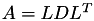or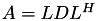CTLDU Computes LDU factorisation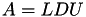CTLL Computes Cholesky factorisation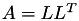or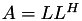►CTLockable Base class for all mutex equipped classes ►CTLowRankApx Base class for all low rank approximation techniques CTLU Computes LU factorisation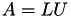►CTMatBuilder Base class for building matrices implementing basic management and parallel construction CTMatrixHierarchy Represents a level-wise hierarchy of matrices ►CTMatrixIO Base class for Matrix IO defining interface ►CTMatrixNorm Baseclass for matrix norm computations ►CTMatrixVis Base class for matrix visualisation CTMINRES Implements the MINRES iteration CTMutex Wraps default mutices CTGraph::TNodes CTNodeSet Represents a set of nodes by an array CTAlgNDCTBuilder::TOptClusterSize Controls optimal cluster size per tree level CTGeomCTBuilder::TOptClusterSize Controls optimal cluster size per tree level CTProcSet Describes a processor set of continuously numbered processors ►CTProgressBar Base class defining interface CTPSCoordVis Coordinate visualisation in PostScript format Ctri_eval_option_t Determines characteristics of triangular system Ctri_quad_rule_t CTGrid::triangle_t CTRichardson Implements Richardson iteration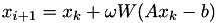CTRowMatrixNorm Computes norm for each row of a matrix CTScopedLock Provides automatic lock and unlock for mutices CTScotchPartStrat Graph partitioning using Scotch CTMatrixHierarchy::TSparseBlockMatrix Represents a n×m block matrix with only a small number of non-null sub matrices stored in an efficient way ►CTTimer Timer class ►CTTruncAcc Defines accuracy for truncation of low rank blocks ►CTTypeInfo Provides basic interface and methods for RTTI ►CTVectorIO Base class for vector IO defining interface CTVRMLCoordVis Coordinate visualisation in VRML format CTVTKCoordVis Coordinate visualisation in VTK format ►Cvector< T > STL class